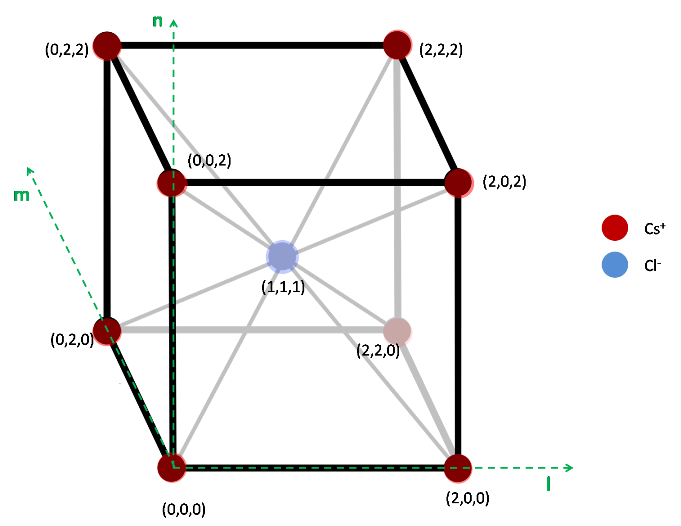#### You may also like### Ball Bearings

If a is the radius of the axle, b the radius of each ball-bearing, and c the radius of the hub, why does the number of ball bearings n determine the ratio c/a? Find a formula for c/a in terms of n.### Air Routes

Find the distance of the shortest air route at an altitude of 6000 metres between London and Cape Town given the latitudes and longitudes. A simple application of scalar products of vectors.### Over-booking

The probability that a passenger books a flight and does not turn up is 0.05. For an aeroplane with 400 seats how many tickets can be sold so that only 1% of flights are over-booked?

# Crystal Symmetry

##### Age 16 to 18Challenge Level

Initially you may find it hard to picture the lattice structure from the given information. The diagram of the body centred cubic lattice below should hopefully aid in your understanding: note how it fulfils the criteria for the integer lattice.Consider the first matrix: $\mathbf{M} = \begin{pmatrix} 1 & 0 & 0 \\ 0 & 0 & -1 \\ 0 & 1& 0 \end{pmatrix}$

Multiplying the each of the unit vectors shows how the axes l, m and n are transformed:

$\mathbf{M} \begin{pmatrix} 1 \\ 0 \\ 0 \end{pmatrix} = \begin{pmatrix} 1 \\ 0 \\ 0 \end{pmatrix}$
$\mathbf{M} \begin{pmatrix} 0 \\ 1 \\ 0 \end{pmatrix} = \begin{pmatrix} 0 \\ 0 \\ 1 \end{pmatrix}$
$\mathbf{M} \begin{pmatrix} 0 \\ 0 \\ 1 \end{pmatrix} = \begin{pmatrix} 0 \\ -1 \\ 0 \end{pmatrix}$

Thus, the l axis is unchanged, but the m axis is rotated onto the n axis, and the n axis onto the negative m axis. In essence this is a rotation of 90$^\circ$ about the l axis in a right-handed sense. It can be easily imagined that every Caesium ion maps straight to another Caesium ion, and that every Chloride ion maps to another chloride ion. Therefore, the crystal structure is preserved.

Now consider the second matrix : $\mathbf{M} = \begin{pmatrix} 3 & 0 & 0 \\ 0 & 1 & 0 \\ 0 & 0 & 2 \end{pmatrix}$

Having already done one matrix transformation, we now recognise that the first column of the matrix is what the unit vector along the l axis is tranformed onto, the second column for the m axis and the third column for the n axis.

Therefore, this transformation represents a stretch of scale factor 3 along the l axis, and 2 along the n axis. This clearly does not preserve the structure of the lattice as the ions are now no longer evenly spaced along each axis!

Consider the third matrix: $\mathbf{M} = \begin{pmatrix} 1 & -1 & 1 \\ 1 & 1 & -1 \\ -1 & 1 & 1 \end{pmatrix}$

From the previous two examples, we have reasoned that a matrix will only preserve the structure of the lattice exactly if it is a pure rotation matrix, with no stretching. A necessary condition for a matrix to be a rotation (but not sufficient) is for columns of the matrix to be orthonormal. Clearly it can be seen from the matrix that the dot product of any of the columns is not zero, and so the columns cannot be orthogonal. Thus, the matrix cannot be a pure rotation, and so will not leave the lattice preserved exactly.

Consider the first vector: $\mathbf{c} = \begin{pmatrix} 1 \\ 0 \\ 0 \end{pmatrix}$

The addition of this vector to the lattice moves each point by 1 unit on the l axis. Although this will not affect the spacing between the atoms in the lattice, it will cause a misaligned, as the first plane of caesium atoms will now be inline with where the first plane of chloride ions were; the first plane of chloride ions will not be in line with where the second plane of caesium ions were etc.

Consider the second vector: $\mathbf{c} = \begin{pmatrix} 1 \\ 1 \\ 0 \end{pmatrix}$

This vector moves the caesium ion at the origin to the position (1, 1, 0) where there was not an ion previously. Therefore, although the spacing in the lattice is preserved, its displacement is noticeable, and so the structure is not completely preserved.

Consider the third vector: $\mathbf{c} = \begin{pmatrix} 2 \\ 2 \\ 2 \end{pmatrix}$

This vector corresponds to caesium ions being moved into the place of another caesium ion (diagonally opposed), and chloride ions doing the same. Thus, the structure is indistinguishable from before, and so is preserved.

Consider the fourth vector: $\mathbf{c} = \begin{pmatrix} 4 \\ -2 \\ -8 \end{pmatrix}$

It can quickly be seen that this translation preserves the lattice since the unit cube of the lattice is of side 2, and that the vector is causing a translation along each of the axes by a multiple of two. Thus caesium ions will always map onto caesium ions, and chloride ions on chloride ions. Thus the lattice will be preserved.

The types of transformation which leave the crystal invariant are:
- Translations using vectors that have components which are multiples of two. This way, caesium ions will always map onto caesium ions and chloride ions onto chloride ions.
- Rotations using matrices. Rotation matrices must have orthonormal columns and must have a determinant of 1.